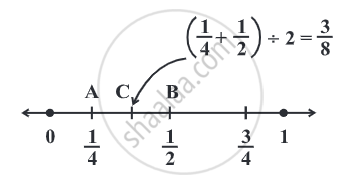# Find a rational number between 14and12. - Mathematics

Sum

Find a rational number between 1/4 "and" 1/2.

#### Solution

(1/4 + 1/2) ÷ 2 = ((1 + 2)/4) ÷ 2 = 3/4 xx 1/2 = 3/8.

3/8 "lies between" 1/4 "and" 1/2.We find the midpoint of AB which is C, represented by
(1/4 + 1/2) ÷ 2 =  3/8.
1/4 < 3/8 < 1/2.
Concept: Rational Numbers Between Two Rational Numbers
Is there an error in this question or solution?